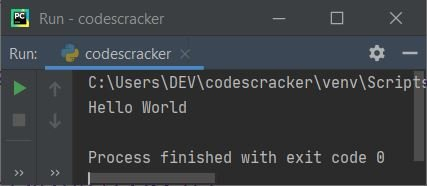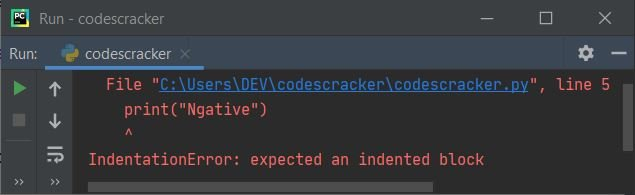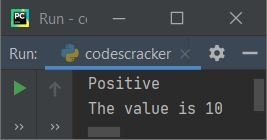# Python Basic Syntax

The basic syntax of a Python program means there are rules to define the correct structure of statements and expressions used in that program. Therefore, in this section, we look up the basic structure of a Python program, or how a Python program can be written to produce effective output.

Unlike most other programming languages like CC++, Java, etc., Python provides a simple syntax to work on. That is, to print "Hello World" in Python, we need to write only this single statement:

`print("Hello World")`

It should be noted that a semicolon is not required at the end of the statement. This is the very simple structure of Python.

Since I've written the above program in the PyCharm IDE. Therefore, after executing the above program, here is the output produced:The output will be "Hello World" regardless of which IDE you use.

While learning about the basic syntax of Python or the basic structure of a Python program, the main thing to look up is the indentation of the program. So let's talk about it.

### Indentation is the most important

Now the question is, what is indentation?
The answer is that "indentation" refers to the spaces available or given at the beginning of a statement in a Python program. Before going deep into it, let's take a look at the program given below:

```val = 10
if val>0:
print("Positive")
elif val<0:
print("Negative")```

The above program can also be written as:

```val = 10
if val>0:
print("Positive")
elif val<0:
print("Negative")```

Both the programs produce the same output, which is:Note: The number of spaces to give before the statement is up to you, the programmer, but the statement needs to be in the same block of code.

The program given below produces the error:

```val = 10
if val>0:
print("Positive")
elif val<0:
print("Negative")```

The error produced by the above Python program is only due to its indentation, as shown in the snapshot given below:The following program also produces an indentation error:

```val = 10
if val>10:
print("Positive")
print("The value is", val)```

The above program should be structured in this way:

```val = 10
if val>0:
print("Positive")
print("The value is", val)```

The output produced by the above program is:Note: Whatever the space you provide, the space must be the same for the same block of code.

You can refer to "get input from the user in Python" to understand the step-by-step process of how different types of inputs can be processed in the Python programming language.

#### More Examples

Python Online Test

« Previous Tutorial Next Tutorial »